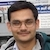# NAEST 2018 Screening Test: Bernoulli's EquationBy

In screening round of National Anveshika Experimental Skills Test ( NAEST 2018 ), a question was asked on the equation of continuity and application of Bernoulli's equation. In NAEST 2016 and NAEST 2015 questions were asked on the same concept.

## Question from NAEST 2018

Question: The water is falling down as stream from a tap. The points A and B are at centre of the water stream. The point A is at a height $h$ above point B. The pressures at A and B are $p_A$ and $p_B$, respectively. The flow speeds at these points are $v_A$ and $v_B$. The density of the water is $\rho$.

1. $p_B < p_A + \rho gh$
2. $p_B = p_A + \rho gh$
3. $v_B = v_A$
4. $v_B > v_A$

Solution: Observe the reduction is the stream's cross-sectional area as it flows down. Let $A_\text{A}$ and $A_\text{B}$ be stream's areas at A and B. Apply continuity equation between A and B to get \begin{align} v_B & =v_A A_\text{A}/A_\text{B} \\ & > v_A\;\; (\text{ because } A_\text{A} > A_\text{B}) \end{align} The flow speed increases as the stream comes down.The flow is steady and streamlined. The conditions for the Bernoulli's equation can be assumed as true. Apply Bernoulli's equation on a streamline through A and B to get \begin{align} p_a+\frac{1}{2}\rho v_A^2+\rho g h= p_B+\frac{1}{2}\rho v_B^2 \end{align} which gives \begin{align} p_B&=p_A+\rho g h -\frac{1}{2}\rho (v_B^2-v_A^2) \\ &< p_A+\rho g h\;\; (\text{ because } v_B>v_A) \end{align} Note that $p_B=p_A+\rho g h$ only when fluid is static (hydrostatic condition). In this case, the water stream is exposed to the atmosphere. The pressures at A and B are approximately equal to the atmospheric pressure.

## Question from NAEST 2016

Question: The height between points A and B is $H$. The pressure of water is $P$, the density of water is $\rho$ and acceleration due to gravity is $g$. Choose the correct option(s)

1. $P_B - P_A < H\rho g$
2. $P_B - P_A = H \rho g$
3. $P_B - P_A > H\rho g$
4. Rate of change of water in gm/sec at point A is equal to rate at point B.

Answer: This question is similar to this NAEST 2018 question. Options (A) and (D) are correct. The volume flow rate is constant in an in-compressible flow.

## Question from NAEST 2015

Question: Two bottles are cut at the base and two straws of equal diameters but different lengths are fitted in the bottle's cap. The bottles are filled with water after closing the straw openings. The straws are opened simultaneously. The water streams start coming out of the bottles. Why bottle with a longer straw gets emptied first?

Answer: Let the difference in lengths of two straws be $y$. The height of water column for the bottle with shorter straw is $h$ and that for the bottle with long straw is $h_y$. Apply Bernoulli's equation to get the velocities of efflux for the two bottles \begin{align} v_1&=\sqrt{2g(h+y)}\;\; \text{ : long straw} \\ v_2&=\sqrt{2gh}\;\;\; \text{ : short straw} \\ \end{align} Thus, the speed of efflux is more for the bottle fitted with long straw. The area of cross-section for the two straws are equal. Hence, the volume flow rate is more for the bottle fitted with longer straw. Hence, this bottle gets emptied first.

We encourage you to find the time at which the bottle gets empty. You are free to make appropriate approximations and a simplified model of the bottle.